4 Displaying Lists
ListPlot is one way to display, or visualize, a list of numbers. There are lots of others. Different ones tend to emphasize different features of a list.
ListLinePlot plots a list, joining up values:
 In:=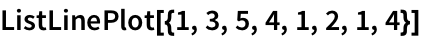Out=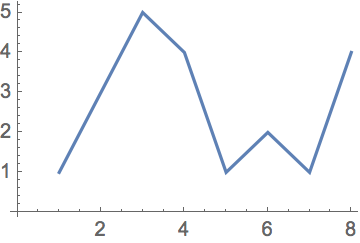When values jump around, its usually harder to understand if you dont join them up:
 In:=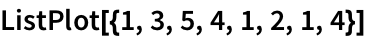Out=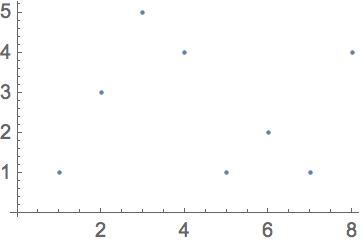In:=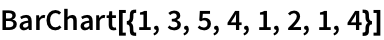Out=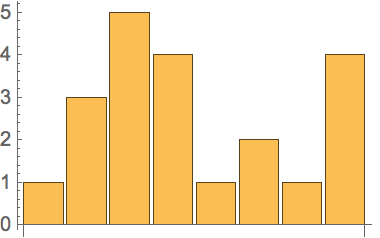In:=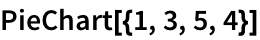Out=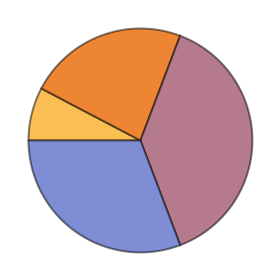In:=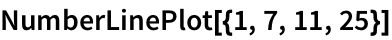Out=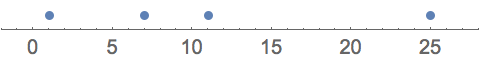In:=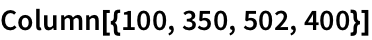Out=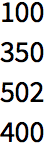Lists can contain anything, including graphics. So you can combine plots by putting them in lists.
Make a list of two pie charts:
 In:=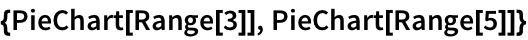Out=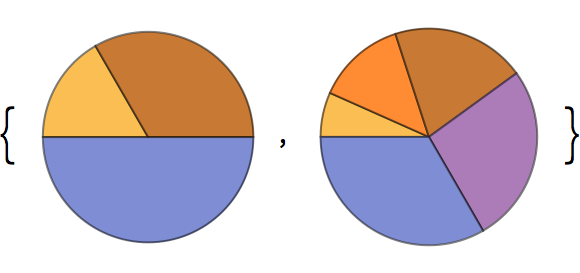Show three bar charts together:
 In:=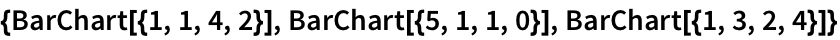Out=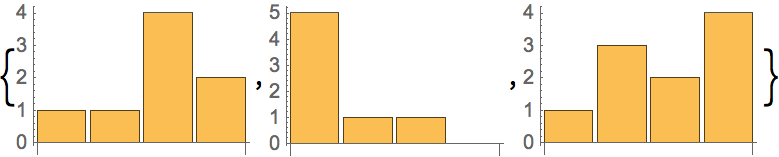4.1Make a bar chart of {1, 1, 2, 3, 5}»
Expected output:
 Out[]=Answer & check your solution
4.2Make a pie chart of numbers from 1 to 10. »
Expected output:
 Out[]=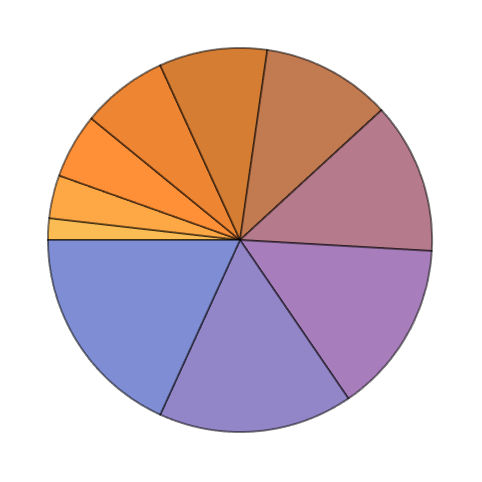Answer & check your solution
4.3Make a bar chart of numbers counting down from 20 to 1. »
Expected output:
 Out[]=Answer & check your solution
4.4Display numbers from 1 to 5 in a column. »
Expected output:
 Out[]=Answer & check your solution
4.5Make a number line plot of the squares {1, 4, 9, 16, 25}»
Expected output:
 Out[]=Answer & check your solution
4.6Make a pie chart with 10 identical segments, each of size 1. »
Expected output:
 Out[]=Answer & check your solution
4.7Make a column of pie charts with 1, 2 and 3 identical segments. »
Expected output:
 Out[]=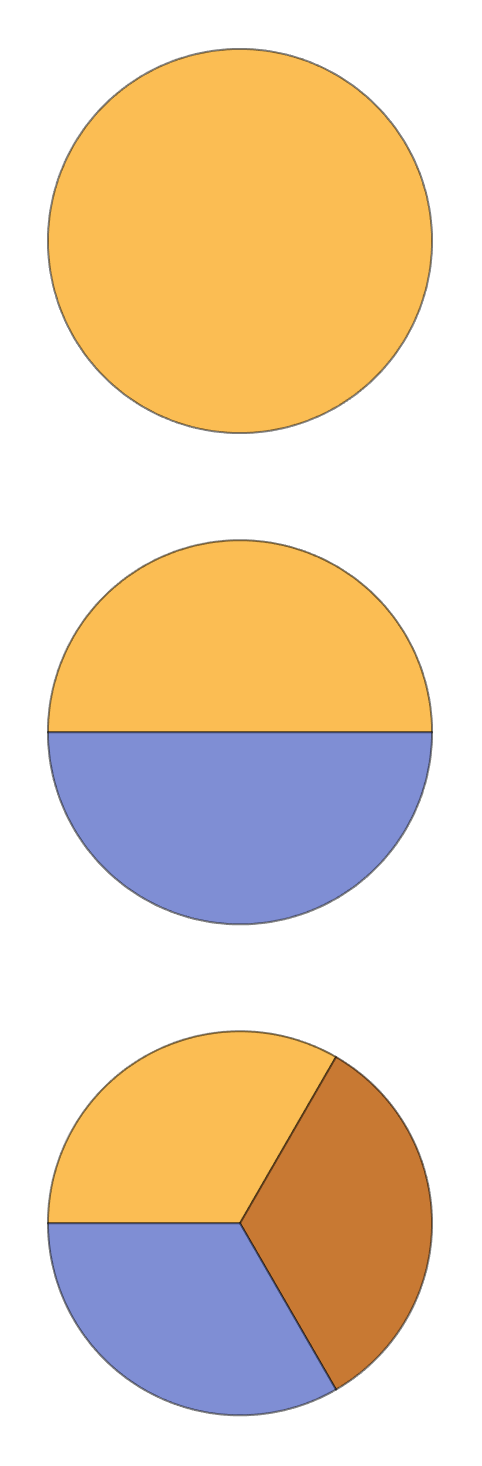Answer & check your solution
+4.1Make a list of pie charts with 1, 2 and 3 identical segments. »
Expected output:
 Out[]=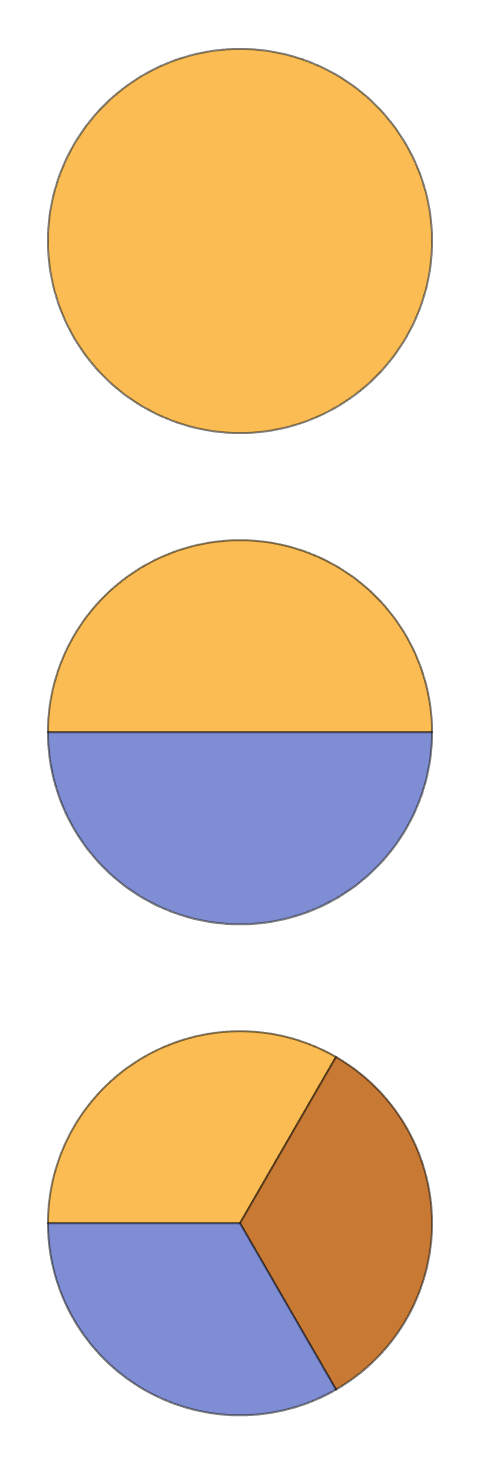Answer & check your solution
+4.2Make a bar chart of the sequence 1, 2, 3, ..., 9, 10, 9, 8, 7, ..., 1. »
Expected output:
 Out[]=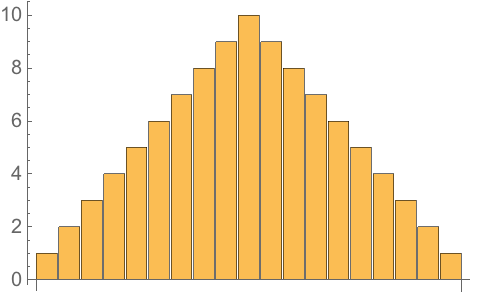Answer & check your solution
+4.3Make a list of a pie chart, bar chart and line plot of the numbers from 1 to 10. »
Expected output:
 Out[]=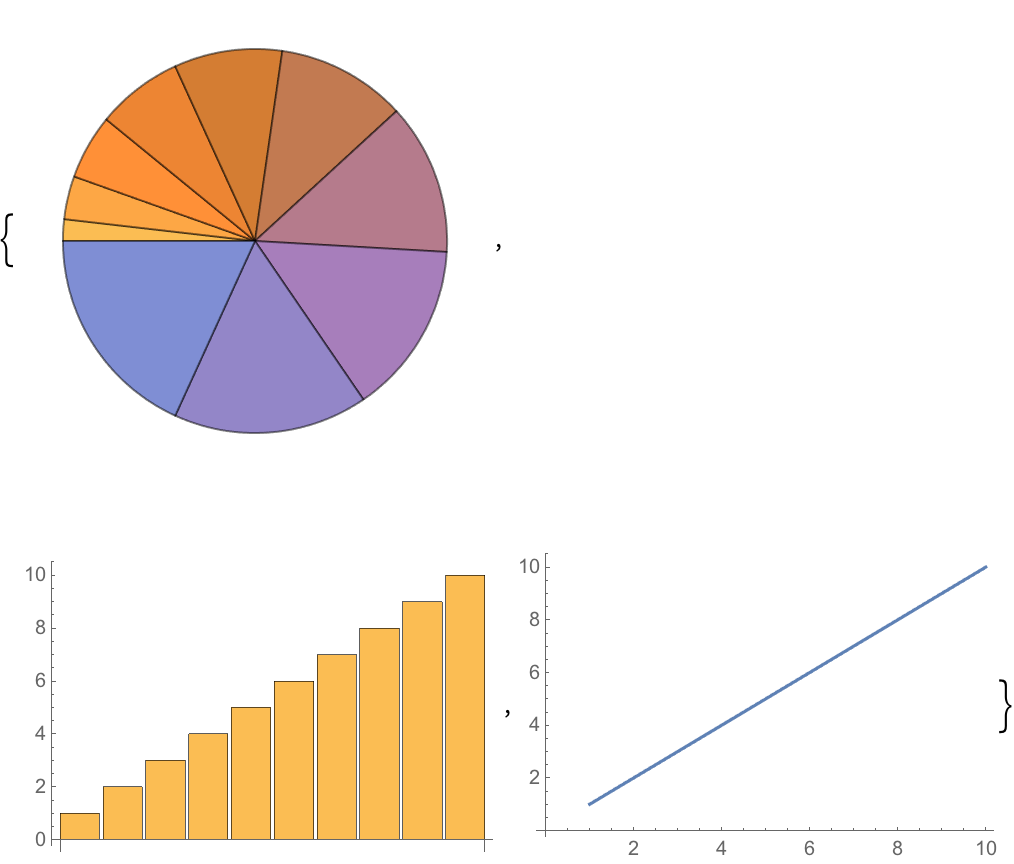Answer & check your solution
+4.4Make a list of a pie chart and a bar chart of {1, 1, 2, 3, 5, 8, 13, 21, 34, 55}»
Expected output:
 Out[]=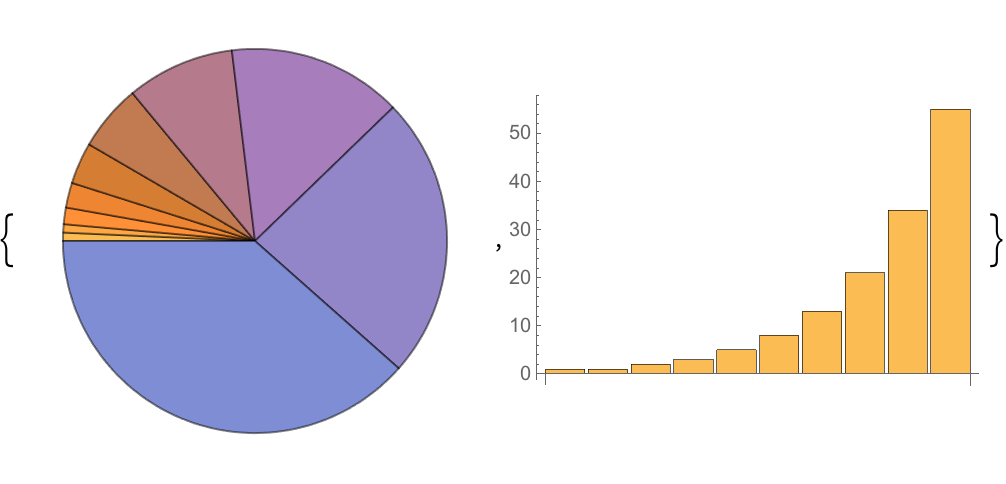Answer & check your solution
+4.5Make a column of two number line plots of {1, 2, 3, 4, 5}»
Expected output:
 Out[]=Answer & check your solution
+4.6Make a number line of fractions 1/2, 1/3, ... through 1/9. »
Expected output:
 Out[]=Answer & check your solution
How do pie charts work in the Wolfram Language?
As in any pie chart, the wedges have relative sizes determined by the relative sizes of numbers in the list. In the Wolfram Language, the wedge for the first number starts at the 9 oclock position, and then subsequent wedges read clockwise. The colors of the wedges are chosen in a definite sequence.
How is the vertical scale determined on plots?
Its set up to automatically include all points except distant outliers. Later on (Section 20), well talk about the PlotRange option, which lets you specify the exact range of the plot.